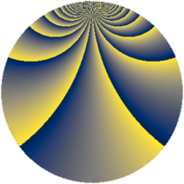# Properties

 Label 192.2.sLevel $192$ Weight $2$ Character orbit 192.s Rep. character $\chi_{192}(11,\cdot)$ Character field $\Q(\zeta_{16})$ Dimension $240$ Newform subspaces $1$ Sturm bound $64$ Trace bound $0$

# Related objects

## Defining parameters

 Level: $$N$$ $$=$$ $$192 = 2^{6} \cdot 3$$ Weight: $$k$$ $$=$$ $$2$$ Character orbit: $$[\chi]$$ $$=$$ 192.s (of order $$16$$ and degree $$8$$) Character conductor: $$\operatorname{cond}(\chi)$$ $$=$$ $$192$$ Character field: $$\Q(\zeta_{16})$$ Newform subspaces: $$1$$ Sturm bound: $$64$$ Trace bound: $$0$$

## Dimensions

The following table gives the dimensions of various subspaces of $$M_{2}(192, [\chi])$$.

Total New Old
Modular forms 272 272 0
Cusp forms 240 240 0
Eisenstein series 32 32 0

## Trace form

 $$240q - 8q^{3} - 16q^{4} - 8q^{6} - 16q^{7} - 8q^{9} + O(q^{10})$$ $$240q - 8q^{3} - 16q^{4} - 8q^{6} - 16q^{7} - 8q^{9} - 16q^{10} - 8q^{12} - 16q^{13} - 8q^{15} - 16q^{16} - 8q^{18} - 16q^{19} - 8q^{21} - 16q^{22} - 48q^{24} - 16q^{25} - 8q^{27} - 16q^{28} - 88q^{30} - 32q^{31} - 16q^{34} - 88q^{36} - 16q^{37} - 8q^{39} - 16q^{40} - 48q^{42} - 16q^{43} - 8q^{45} - 16q^{46} - 8q^{48} - 16q^{49} - 8q^{51} + 32q^{52} - 8q^{54} - 80q^{55} - 8q^{57} + 128q^{58} - 8q^{60} - 16q^{61} + 80q^{64} - 24q^{66} - 144q^{67} - 8q^{69} + 80q^{70} - 8q^{72} - 16q^{73} - 8q^{75} + 96q^{76} + 16q^{78} - 48q^{79} - 8q^{81} + 64q^{82} + 104q^{84} - 16q^{85} - 8q^{87} + 64q^{88} + 136q^{90} - 16q^{91} - 32q^{93} + 80q^{94} + 128q^{96} - 8q^{99} + O(q^{100})$$

## Decomposition of $$S_{2}^{\mathrm{new}}(192, [\chi])$$ into newform subspaces

Label Dim. $$A$$ Field CM Traces $q$-expansion
$$a_2$$ $$a_3$$ $$a_5$$ $$a_7$$
192.2.s.a $$240$$ $$1.533$$ None $$0$$ $$-8$$ $$0$$ $$-16$$